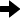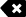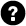код Online Math Calculator - Calculatorology

## Online Math Calculator( ) % x-17 8 9 √ xy 4 5 6 × ÷ 1 2 3 + - 0 . E Ans =

### Online math calculator

The online math calculator is used to perform a variety of calculations. It is comprised of the forward and back controls which allow you to move the cursor in either direction. You can use it to navigate across a long calculations or values in case you want to change the figures. The backspace is used to delete the digits to the left. If you want to erase all data of the previous calculations from the text field, you will use the ‘Clear all’ button designated by a trash bin. You can use the button with the question symbol if you need help with any of the calculator controls.

The undo button is used to undo the last editing change while redo enters the previous editing change. You can select the expression using the control button and letter A. To get the calculation result, you will use the ‘Ans’ button to execute the calculations. The online math calculator uses particular formulas in executing the conversions.

For example, to get the reciprocal of a value, you will click on ‘X-1’. Where, X-1 = 1/x. This calculator contains calculation symbols such as the addition, subtraction, multiplication and division. You can also determine the square root of a value or determine their percentages.

#### For example;

Determine the square root of 81

##### Solution;
You will first click on the square root symbol and then enter 81. Click on the equal sign at the bottom right side of the calculator. Your answer will be displayed as; 9.

This calculator is easier to use and can perform a variety of math calculations. It has a large text field to give you enough space while calculating larger values. It is important to enter the appropriate values when using this calculator since it gives accurate results depending on the input.